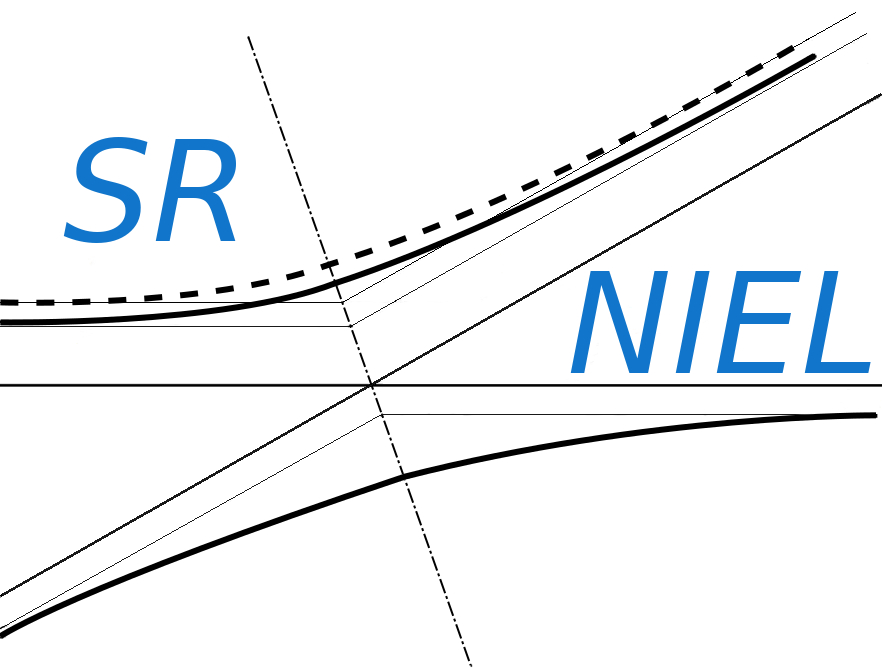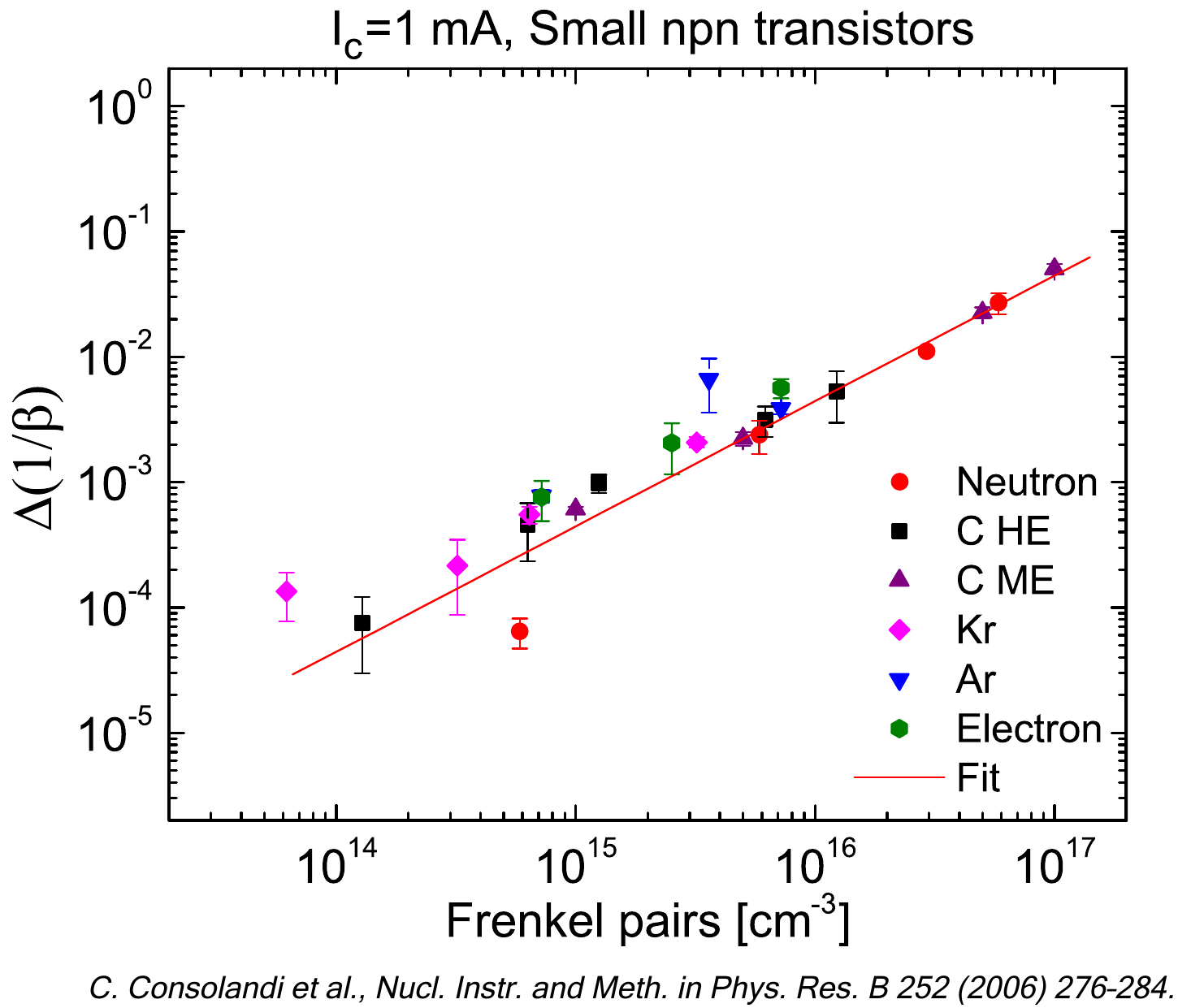SR-NIEL – 7 Screened Relativistic (SR) Treatment for NIEL Dose Nuclear and Electronic Stopping Power Calculator (version 8.0)This is the Non-Ionizing Energy-Loss (NIEL) Code Web application for neutrons.

### How to use this Neutron NIEL Calculator

This tool allows one to obtain the NIEL values and NIEL doses from the tabulated damage functions values found in Table A1.1 (for Si) and Table A2.1 (for GaAs) in ASTM-722 (2009, 2014 and 2019). For information about the computation method take a look at the Neutron Damage Function and NEIL page.

The input parameters and options for the tool are described below. When the input form has been completed, pressing the "CALCULATE" button will start the calculation and open the "Results" page (allow for pop-up in your browser settings). The result page will be also linked at the bottom of the calculator page.

### Input Parameters:

- Damage function
- Target material (Si or GaAs)
- Calculator energy limits
- Particle fluence.

### Damage Function

Here it is possible to select the damage function corresponding to different ASTM-722 (2009, 2014 and 2019)  standards.

### Target Material

In this section it is possible to specify the target material selecting Si or GaAs. The displacement threshold energy will change accordingly (25 eV or 20.5 eV for Si and 10 eV for GaAs).

### Energy Limits

This section define the energy limits of the calculation, and the following parameters will be defined:
- Minimum Energy of the incident particle
- Maximum Energy of the incident particle

The output table will contain The energy fixed values as expressed in ASTM-E722 (2009, 2014 and 2019).

### Particle Fluence for Displacement Damage Dose Calculation

Here is defined the particle fluence that is used to obtain the deposited NIEL Dose (i.e., the displacement damage dose).

### Result

The result page contains the input parameters, the NIEL curve and the result table, which includes the NIEL, and the NIEL Dose (in [MeV g-1] and [Gy])